# Test: Continuous Time Fourier Series

## 8 Questions MCQ Test GATE Electrical Engineering (EE) 2023 Mock Test Series | Test: Continuous Time Fourier Series

Description
Attempt Test: Continuous Time Fourier Series | 8 questions in 30 minutes | Mock test for Electrical Engineering (EE) preparation | Free important questions MCQ to study GATE Electrical Engineering (EE) 2023 Mock Test Series for Electrical Engineering (EE) Exam | Download free PDF with solutions
QUESTION: 1

Solution:
QUESTION: 2

Solution:
QUESTION: 3

### A periodic triangular wave is shown in figure its fourier components will consists only of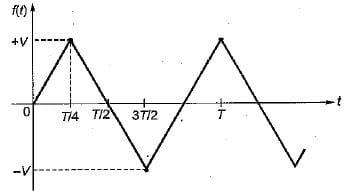Solution:
QUESTION: 4

Determine the Fourier series coefficient for given periodic signal x(t) is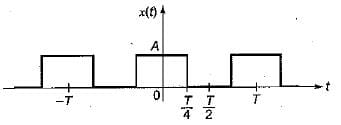Solution: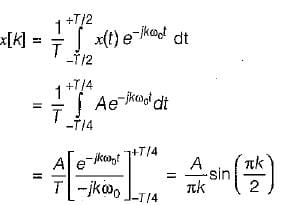QUESTION: 5

Consider the three continuous time signals with fundamental period of T = 1/2
x(t) = cos4πt
y(t) = sin 4πt
z(t) = x(t)·y(t)

The Fourier co-efficient of z(t) are given by

Solution: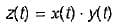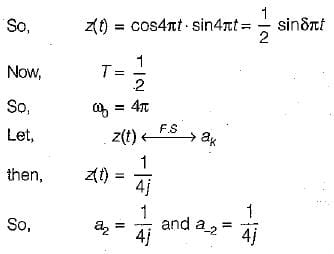QUESTION: 6

Consider a continuous time periodic signal x(t) given by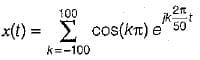Solution: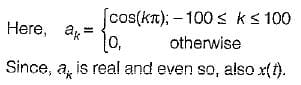QUESTION: 7

The Fourier series coefficient of time domain signal have been given. Determine the corresponding time domain signal and choose correct option.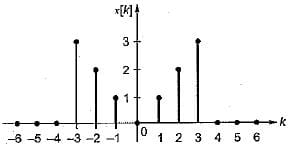Solution: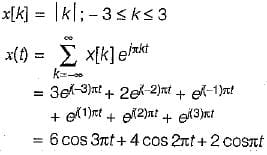QUESTION: 8

If f(x) = | cosx | ; (-π < x < π), then the fourier series coefficient a0 and b2 is

Solution: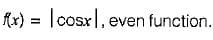∴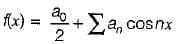Here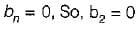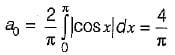Use Code STAYHOME200 and get INR 200 additional OFF Use Coupon Code